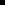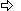### Set Individual Data Values

CimData, the interface for both the X and Y series vectors, has a property called Item.

Item:Allows you to get and set individual data values within the vector.Is the default property of CimData. It can be invoked without having to write its name.Returns a single variant type when retrieving data and expects a single variant type when setting the data.

This variant type can be a single value of any of the following Basic types that result in a numeric value including:IntegerLongSingleDoubleDecimalBooleanByteVariantString

To invoke the Item property to retrieve data you can use either of the following two formats:X = CimData.Item(n)X = CimData(n)

Where

n is the location of the data value you are retrieving.

To invoke the Item property to set data you can use either of the following two formats:CimData.Item(n) = XCimData(n) = X

Where

n is the location of the data value you are setting.

Scripting examples for setting single and array values include:

Example 1. Access Data Values from a Series Vectors

Series name: Series 1

Script purpose: Set a point to the third Y data value

Procedure:

1. Open the CimEdit Properties dialog box for the XY Plot control object.

2. Display the Edit Script tab.

' Define the point:

Dim x As New Point

x.Id ="X_POINT"

' Set the point to the third Y data value in the series:

x.SetValue = cimOleObj.Series("Series 1").Data.Y(2)

Example 2. Set Individual Data Values to a Particular Value

Series name: Series 1

Script purpose: Set the fifth X data value to the value of a point

Procedure:

1. Open the CimEdit Properties dialog box for the XY Plot control object.

2. Display the Edit Script tab.

3. Write the following Basic excerpt within a subroutine.

' Define the point:

Dim x As New Point

x.Id ="X_POINT"

' Set the fifth X (which has an index of 4)

' data value in the series:

cimOleObj.Series("Series 1").Data.X(4) = x.GetValue

Example 3. Set Individual Data Values to Unavailable

Series name: Series 1

Script purpose: Set the fourth y data value to null, the fourth X data value to empty

Both operations result in the value being unavailable.

Procedure:

1. Open the CimEdit Properties dialog box for the XY Plot control object.

2. Display the Edit Script tab.

Write the following Basic excerpt within a subroutine.

' Set the fourth X and Y data values to unavailable

' (either null or empty):

cimOleObj.Series("Series 1").Data.Y(3) = Null

cimOleObj.Series("Series 1").Data.X(3) = Empty

 Scripting examples for XY plots.